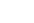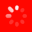The No.1 Website for Pro Audio
All  This Thread  Reviews  Gear Database  Gear for saleLatest  Trending
Here for the gear

Help with equation calculation for helmholtz resonator

Can someone provide a step by step process for calculate helmholtz resonance, if say im trying to calculate the port area instead of the frequency. Im not great with math but I want to learn and understand how to do this without online calculators.

this is the equation I am using.

Cavity Resonance

Also, no neck.
Gear Guru

See if the following program works for you.
mh-audio.nl - Acoustic
Caution they are not a silver bullet.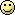Here for the gear

Quote:
See if the following program works for you.
mh-audio.nl - Acoustic
Caution they are not a silver bullet.I guess that you misunderstand. I want to do the math myself.
Gear Guru

Quote:
I guess that you misunderstand. I want to do the math myself.
Totally misread your post.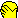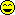Here for the gear

L= Length of neck
Vo = Volume
A = Area of port
F = frequency obviously

L = 0
Vo = 473.17 (I think its cubed though I calculated it from 16 ounces, so it should be cubed right??)
A = What im solving for
F = 480 hz
V= speed of sound which is 340.19 at sea level

If Vo needs to be cubed then A should be squared, but I dont know if Im supposed to do that

Ive put all this down on paper and im not sure how to run this equation to solve especially when it comes to the square root of a fraction, especially if the fraction is cubed and squared.

How do I do this??? Been searching the internet for 2 days now trying to figure this out.
Lives for gear

Even if you want to do math I would still recommend to download "AcousticCalculator"(windows) from mh-audio.nl It will be much easier...
Here for the gear

SoS = speed of sound at the approximated temperature

F = (SoS/2pi) * √(A / VL)

V = A / (L(F/(SoS/2pi)))^2

L = A / (V(F/(SoS/2pi)))^2

(VL) * (F/(SoS/2pi))^2 = A

Excel

C5 = area, C4 = length, C7 = frequency, C6 = The speed of sound

V=SUM(C5/(C4*(C7/(C6/(2*(22/7))))^2))

D5 = area, D3 = area, D7 = frequency, D6 = The speed of sound

L=SUM(D5/(D3*(D7/(D6/(2*(22/7))))^2))

E3 = area, E4 = length, E7 = frequency, E6 = The speed of sound

A=SUM(E3*E4) * (E7/(E6/(2*(22/7))))^2

Quote:
Can someone provide a step by step process for calculate helmholtz resonance, if say im trying to calculate the port area instead of the frequency. Im not great with math but I want to learn and understand how to do this without online calculators.

this is the equation I am using.

Cavity Resonance

Also, no neck.

Welcome to the Gearslutz Pro Audio Community!

Registration benefits include:
• The ability to reply to and create new discussions
• Access to members-only giveaways & competitions
• Interact with VIP industry experts in our guest Q&As
• Access to members-only sub forum discussions
• Get INSTANT ACCESS to the world's best private pro audio Classifieds for only USD \$20/year
• Promote your eBay auctions and Reverb.com listings for free
• Remove this message!
You need an account to post a reply. Create a username and password below and an account will be created and your post entered.